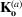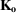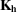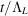International Tables for Crystallography (2006). Vol. B. ch. 5.1, pp. 534-551   | 1 | 2 | https://doi.org/10.1107/97809553602060000569

## Contents

• 5.1. Dynamical theory of X-ray diffraction  (pp. 534-551)
• 5.1.1. Introduction  (p. 534) | html | pdf |
• 5.1.2. Fundamentals of plane-wave dynamical theory  (pp. 534-538) | html | pdf |
• 5.1.2.1. Propagation equation  (pp. 534-535) | html | pdf |
• 5.1.2.2. Wavefields  (pp. 535-536) | html | pdf |
• 5.1.2.3. Boundary conditions at the entrance surface  (p. 536) | html | pdf |
• 5.1.2.4. Fundamental equations of dynamical theory  (p. 536) | html | pdf |
• 5.1.2.5. Dispersion surface  (pp. 536-537) | html | pdf |
• 5.1.2.6. Propagation direction  (pp. 537-538) | html | pdf |
• 5.1.3. Solutions of plane-wave dynamical theory  (pp. 538-541) | html | pdf |
• 5.1.3.1. Departure from Bragg's law of the incident wave  (p. 538) | html | pdf |
• 5.1.3.2. Transmission and reflection geometries  (pp. 538-539) | html | pdf |
• 5.1.3.3. Middle of the reflection domain  (p. 539) | html | pdf |
• 5.1.3.4. Deviation parameter  (p. 539) | html | pdf |
• 5.1.3.5. Pendellösung and extinction distances  (pp. 539-540) | html | pdf |
• 5.1.3.6. Solution of the dynamical theory  (p. 540) | html | pdf |
• 5.1.3.7. Geometrical interpretation of the solution in the zero-absorption case  (pp. 540-541) | html | pdf |
• 5.1.3.7.1. Transmission geometry  (p. 540) | html | pdf |
• 5.1.3.7.2. Reflection geometry  (p. 541) | html | pdf |
• 5.1.4. Standing waves  (p. 541) | html | pdf |
• 5.1.5. Anomalous absorption  (p. 541) | html | pdf |
• 5.1.6. Intensities of plane waves in transmission geometry  (pp. 541-545) | html | pdf |
• 5.1.6.1. Absorption coefficient  (pp. 541-542) | html | pdf |
• 5.1.6.2. Boundary conditions for the amplitudes at the entrance surface – intensities of the reflected and refracted waves  (p. 542) | html | pdf |
• 5.1.6.3. Boundary conditions at the exit surface  (pp. 542-543) | html | pdf |
• 5.1.6.3.1. Wavevectors  (pp. 542-543) | html | pdf |
• 5.1.6.3.2. Amplitudes – Pendellösung   (p. 543) | html | pdf |
• 5.1.6.4. Reflecting power  (pp. 543-544) | html | pdf |
• 5.1.6.5. Integrated intensity  (pp. 544-545) | html | pdf |
• 5.1.6.5.1. Non-absorbing crystals  (pp. 544-545) | html | pdf |
• 5.1.6.5.2. Absorbing crystals  (p. 545) | html | pdf |
• 5.1.6.6. Thin crystals – comparison with geometrical theory  (p. 545) | html | pdf |
• 5.1.7. Intensity of plane waves in reflection geometry  (pp. 545-548) | html | pdf |
• 5.1.7.1. Thick crystals  (pp. 545-546) | html | pdf |
• 5.1.7.1.1. Non-absorbing crystals  (pp. 545-546) | html | pdf |
• 5.1.7.1.2. Absorbing crystals  (p. 546) | html | pdf |
• 5.1.7.2. Thin crystals  (pp. 546-548) | html | pdf |
• 5.1.7.2.1. Non-absorbing crystals  (pp. 546-547) | html | pdf |
• 5.1.7.2.2. Absorbing crystals  (pp. 547-548) | html | pdf |
• 5.1.8. Real waves  (pp. 548-550) | html | pdf |
• 5.1.8.1. Introduction  (p. 548) | html | pdf |
• 5.1.8.2. Borrmann triangle  (pp. 548-549) | html | pdf |
• 5.1.8.3. Spherical-wave Pendellösung   (pp. 549-550) | html | pdf |
• Appendix 5.1.1.   (pp. 550-551) | html | pdf |
• A5.1.1.1. Dielectric susceptibility – classical derivation  (p. 550) | html | pdf |
• A5.1.1.2. Maxwell's equations  (pp. 550-551) | html | pdf |
• A5.1.1.3. Propagation equation  (p. 551) | html | pdf |
• A5.1.1.4. Poynting vector  (p. 551) | html | pdf |
• References | html | pdf |
• Figures
• Fig. 5.1.2.1. Bragg reflection  (p. 535) | html | pdf |
• Fig. 5.1.2.2. Boundary condition for wavevectors at the entrance surface of the crystal  (p. 536) | html | pdf |
• Fig. 5.1.2.3. Intersection of the dispersion surface with the plane of incidence  (p. 537) | html | pdf |
• Fig. 5.1.2.4. Intersection of the dispersion surface with the plane of incidence shown in greater detail  (p. 537) | html | pdf |
• Fig. 5.1.2.5. Dispersion surface for the two states of polarization  (p. 538) | html | pdf |
• Fig. 5.1.3.1. Departure from Bragg's law of an incident wave  (p. 538) | html | pdf |
• Fig. 5.1.3.2. Transmission, or Laue, geometry  (p. 538) | html | pdf |
• Fig. 5.1.3.3. Reflection, or Bragg, geometry  (p. 539) | html | pdf |
• Fig. 5.1.3.4. Boundary conditions at the entrance surface for transmission geometry  (p. 539) | html | pdf |
• Fig. 5.1.3.5. Boundary conditions at the entrance surface for reflection geometry  (p. 540) | html | pdf |
• Fig. 5.1.6.1. Variation of the effective absorption with the deviation parameter in the transmission case for the 400 reflection of GaAs using Cu K α radiation  (p. 542) | html | pdf |
• Fig. 5.1.6.2. Variation of the intensities of the reflected and refracted waves in an absorbing crystal for the 220 reflection of Si using Mo K α radiation, t = 1 mm (μ t = 1.42)  (p. 542) | html | pdf |
• Fig. 5.1.6.3. Boundary condition for the wavevectors at the exit surface  (p. 543) | html | pdf |
• Fig. 5.1.6.4. Decomposition of a wavefield into its two components when it reaches the exit surface  (p. 543) | html | pdf |
• Fig. 5.1.6.5. Cross sections of the incident,, refracted,, and reflected,, waves  (p. 544) | html | pdf |
• Fig. 5.1.6.6. Theoretical rocking curves in the transmission case for non-absorbing crystals and for various values of(p. 544) | html | pdf |
• Fig. 5.1.6.7. Variations with crystal thickness of the integrated intensity in the transmission case (no absorption) (arbitrary units)  (p. 545) | html | pdf |
• Fig. 5.1.7.1. Theoretical rocking curve in the reflection case for a non-absorbing thick crystal in terms of the deviation parameter  (p. 545) | html | pdf |
• Fig. 5.1.7.2. Theoretical rocking curve in the reflection case for a thick absorbing crystal  (p. 546) | html | pdf |
• Fig. 5.1.7.3. Bragg case: thick crystals  (p. 546) | html | pdf |
• Fig. 5.1.7.4. Bragg case: thin crystals  (p. 547) | html | pdf |
• Fig. 5.1.8.1. Borrmann triangle  (p. 548) | html | pdf |
• Fig. 5.1.8.2. Packet of wavefields of divergence Δα excited in the crystal by an incident wavepacket of angular width(p. 549) | html | pdf |
• Fig. 5.1.8.3. Intensity distribution along the base of the Borrmann triangle  (p. 549) | html | pdf |
• Fig. 5.1.8.4. Interference at the origin of the Pendellösung fringes in the case of an incident spherical wave  (p. 550) | html | pdf |
• Fig. 5.1.8.5. Spherical-wave Pendellösung fringes observed on a wedge-shaped crystal  (p. 550) | html | pdf |